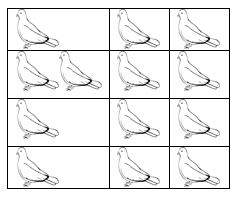# Mathematics | The Pigeonhole Principle

Suppose that a flock of 20 pigeons flies into a set of 19 pigeonholes to roost. Because there are 20 pigeons but only 19 pigeonholes, a least one of these 19 pigeonholes must have at least two pigeons in it. To see why this is true, note that if each pigeonhole had at most one pigeon in it, at most 19 pigeons, one per hole, could be accommodated. This illustrates a general principle called the pigeonhole principle, which states that if there are more pigeons than pigeonholes, then there must be at least one pigeonhole with at least two pigeons in it.Theorem –

I) If “A” is the average number of pigeons per hole, where A is not an integer then

• At least one pigeon hole contains ceil[A] (smallest integer greater than or equal to A) pigeons
• Remaining pigeon holes contains at most floor[A] (largest integer less than or equal to A) pigeons

Or

II) We can say as, if n + 1 objects are put into n boxes, then at least one box contains two or more objects.
The abstract formulation of the principle: Let X and Y be finite sets and letbe a function.

• If X has more elements than Y, then f is not one-to-one.
• If X and Y have the same number of elements and f is onto, then f is one-to-one.
• If X and Y have the same number of elements and f is one-to-one, then f is onto.

Pigeonhole principle is one of the simplest but most useful ideas in mathematics. We will see more applications that proof of this theorem.

• Example – 1: If (Kn+1) pigeons are kept in n pigeon holes where K is a positive integer, what is the average no. of pigeons per pigeon hole?

Solution: average number of pigeons per hole = (Kn+1)/n
= K + 1/n
Therefore at least a pigeonholes contains (K+1) pigeons i.e., ceil[K +1/n] and remaining contain at most K i.e., floor[k+1/n] pigeons.
i.e., the minimum number of pigeons required to ensure that at least one pigeon hole contains (K+1) pigeons is (Kn+1).

• Example – 2: A bag contains 10 red marbles, 10 white marbles, and 10 blue marbles. What is the minimum no. of marbles you have to choose randomly from the bag to ensure that we get 4 marbles of same color?

Solution: Apply pigeonhole principle.
No. of colors (pigeonholes) n = 3
No. of marbles (pigeons) K+1 = 4
Therefore the minimum no. of marbles required = Kn+1
By simplifying we get Kn+1 = 10.
Verification: ceil[Average] is [Kn+1/n] = 4
[Kn+1/3] = 4
Kn+1 = 10
i.e., 3 red + 3 white + 3 blue + 1(red or white or blue) = 10

Pigeonhole principle strong form –

Theorem: Let q1, q2, . . . , qn be positive integers.
If q1+ q2+ . . . + qn – n + 1 objects are put into n boxes, then either the 1st box contains at least q1 objects, or the 2nd box contains at least q2 objects, . . ., the nth box contains at least qn objects.

Application of this theorem is more important, so let us see how we apply this theorem in problem solving.

• Example – 1: In a computer science department, a student club can be formed with either 10 members from first year or 8 members from second year or 6 from third year or 4 from final year. What is the minimum no. of students we have to choose randomly from department to ensure that a student club is formed?

Solution: we can directly apply from the above formula where,
q1 =10, q2 =8, q3 =6, q4 =4 and n=4
Therefore the minimum number of students required to ensure department club to be formed is
10 + 8 + 6 + 4 – 4 + 1 = 25

• Example – 2: A box contains 6 red, 8 green, 10 blue, 12 yellow and 15 white balls. What is the minimum no. of balls we have to choose randomly from the box to ensure that we get 9 balls of same color?

Solution: Here in this we cannot blindly apply pigeon principle. First we will see what happens if we apply above formula directly.
From the above formula we have get answer 47 because 6 + 8 + 10 + 12 + 15- 5 + 1 = 47
But it is not correct. In order to get the correct answer we need to include only blue, yellow and white balls because red and green balls are less than 9. But we are picking randomly so we include after we apply pigeon principle.
i.e., 9 blue + 9 yellow + 9 white – 3 + 1 = 25
Since we are picking randomly so we can get all the red and green balls before the above 25 balls. Therefore we add 6 red + 8 green + 25 = 39
We can conclude that in order to pick 9 balls of same color randomly, one has to pick 39 balls from a box.

References:
http://www2.fiit.stuba.sk/~kvasnicka/Mathematics%20for%20Informatics/Rosen_Discrete_Mathematics_and_Its_Applications_7th_Edition.pdf